# KSEEB Solutions for Class 5 Maths Chapter 5 Fractions

Students can Download Maths Chapter 5 Fractions Questions and Answers, Summary, Notes Pdf, KSEEB Solutions for Class 5 Maths helps you to revise the complete Karnataka State Board Syllabus and score more marks in your examinations.

## Karnataka State Syllabus Class 5 Maths Chapter 5 Fractions

### KSEEB Class 5 Maths Fractions Ex 5.1

KSEEB Solutions For Class 5 Maths Question 1.
Which of the following figures have been divided into parts of the same size?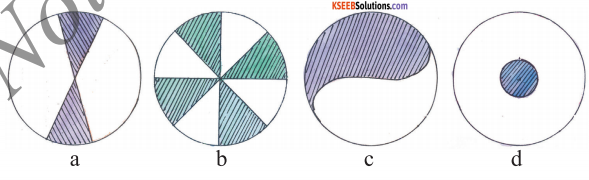b & c.KSEEB Solutions For Class 5 Maths Chapter 5 Question 2.
Draw a line to divide each of these figures into the required number of equal parts.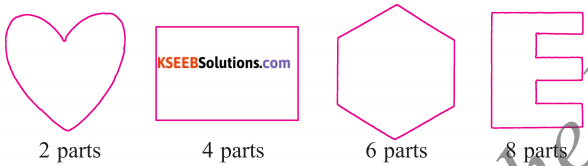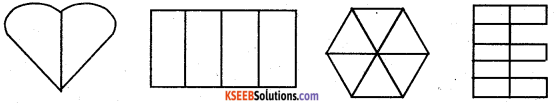Class 5 Maths Chapter 5 Fraction Question 3.
Write each of the following as a fraction
a) Half –$$\frac{1}{2}$$
b) Two-third – $$\frac{2}{3}$$
c) Two-tenth – $$\frac{2}{10}$$
d) Five-seventh – $$\frac{5}{7}$$
e) Five-sixteenth – $$\frac{5}{16}$$
f) Five-twelveth – $$\frac{5}{12}$$
g) Eight-nineth – $$\frac{8}{9}$$
h) Four-nineth – $$\frac{4}{9}$$
i) Three-fourth – $$\frac{3}{4}$$
j) Two-fifth – $$\frac{2}{5}$$KSEEB 5th Maths Solutions Question 4.
Write each of the fraction in words
a) $$\frac{2}{5}$$ – two-fifth
b) $$\frac{3}{4}$$ – three-fourth
c) $$\frac{7}{10}$$ – seven-tenth
d) $$\frac{11}{12}$$ – eleven-twelveth
e) $$\frac{2}{3}$$ – two-third
f) $$\frac{4}{5}$$ – four-fifth
g) $$\frac{5}{8}$$ – five-eight
h) $$\frac{3}{7}$$ – three-seventh
i) $$\frac{5}{6}$$ – five-sixth
j) $$\frac{7}{9}$$ – seven-ninethKSEEB Solutions For 5th Class Maths Question 5.
What fraction of the figure is shaded?
a) $$\frac{2}{3}$$b) $$\frac{2}{4}$$c) $$\frac{5}{8}$$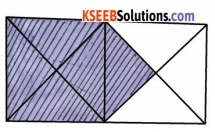d) $$\frac{7}{12}$$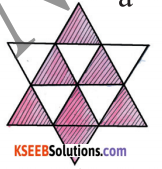e) $$\frac{7}{15}$$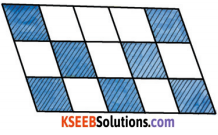f) $$\frac{6}{18}$$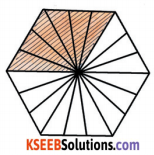KSEEB Solutions 5th Standard Maths Question 6.
Fill in the blanks:
a) The denominator of the fraction $$\frac{1}{8}$$ is 8
b) The numerator of the fraction is $$\frac{2}{5}$$ 2
c) 3 is the denominator of fraction $$\frac{1}{3}$$
d) 1 is the numerater of the fraction $$\frac{1}{5}$$
e) In a fraction, the denominator is written below the numerator separated by a line.KSEEB Solutions Class 5 Maths Question 7.
Write down the fraction of the coloured portions in these figures.
a)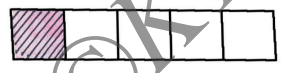$$\frac{1}{5}$$

b)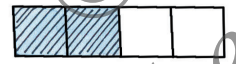$$\frac{2}{4}$$

c)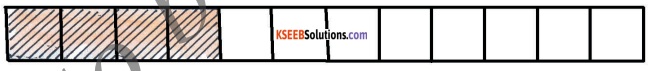$$\frac{4}{12}$$

d)$$\frac{3}{6}$$

e)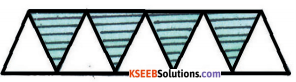$$\frac{4}{9}$$5th Standard Maths Fraction Question 8.
Write the fraction for the shaded part in each of the following figures.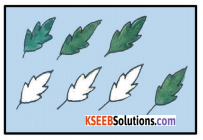$$\frac{4}{7}$$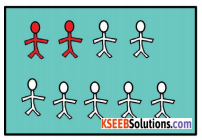$$\frac{2}{9}$$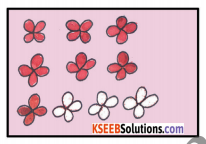$$\frac{7}{10}$$KSEEB Solutions For Class 5th Maths Question 9.
Colour the figures as instructed.

Eg: $$\frac{7}{10}$$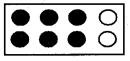$$\frac{1}{5}$$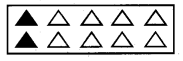$$\frac{2}{3}$$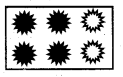$$\frac{2}{9}$$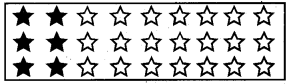KSEEB Class 5 Maths Question 10.
Represent the given fraction by drawing a line.
a) What is $$\frac{1}{3}$$ of 12?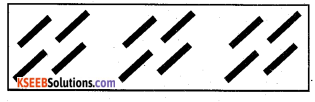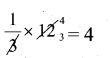b) What is $$\frac{2}{5}$$ of 15?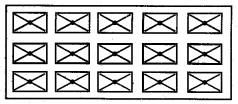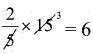c) What is $$\frac{2}{7}$$ of 21?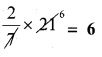5th Standard Fraction Lesson Question 11.
Shade the figures to show the fractions.
a) $$\frac{6}{11}$$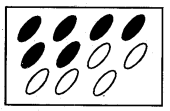b) $$\frac{7}{20}$$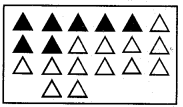c) $$\frac{8}{15}$$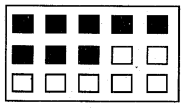5th Standard Maths Notes Question 12.
Which of the following shaded figures represent $$\frac{4}{9}$$ ?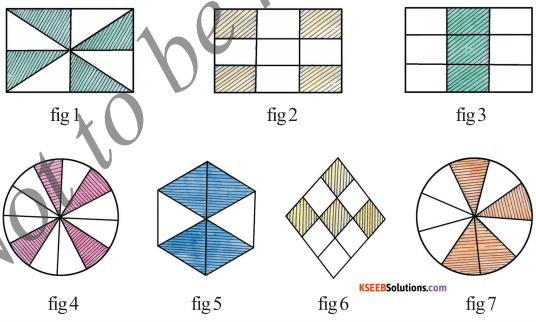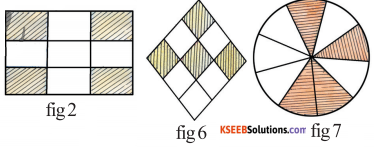5th Class Maths Fractions Question 13.
Which of the following shaded figure represents $$\frac{5}{12}$$ and give reason.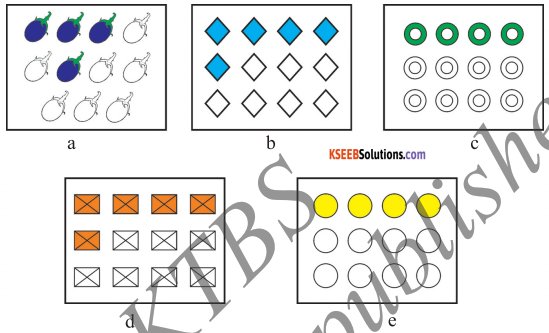fig b&d, Reason: 5 objectives out of 12 are shaded

Fraction Chapter Class 5 Question 14.
Represent the grouped parts as fractions:
a)$$\frac{4}{5}$$

b)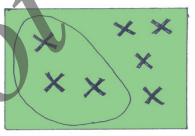$$\frac{3}{7}$$

c)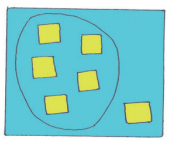$$\frac{5}{6}$$### KSEEB Class 5 Maths Fractions Ex 5.2

I. Fill in the gaps using > or < signs.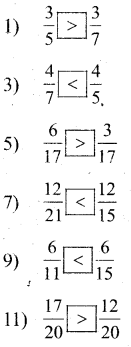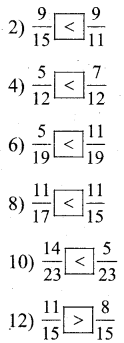### KSEEB Class 5 Maths Fractions Ex 5.3

I. Complete the series.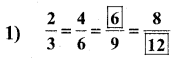2 × 2 = 4
2 × 3 = 6

3 × 2 =6
3 × 3 = 9
3 × 4 = 12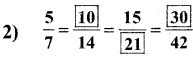5 × 2 = 10
7 × 2 = 14

5 × 3 = 15
7 × 3 = 21

5 × 6 = 30
7 × 6 = 42II. Write the next three equivalent fractions.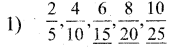2 × 3 = 6, 5 × 3 = 15
2 × 4 = 8, 5 × 4 = 20
2 × 5 = 10, 5 × 5 = 25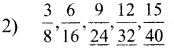3 × 3 = 9
3 × 4 = 12
3 × 5 = 15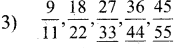9 × 3 = 27, 11 × 3 = 33
9 × 4 = 36, 11 × 4 = 44
9 × 5 = 45, 11 × 5 = 55III. Are the two fractions equivalent?

Karnataka State Class 5 Maths Solutions Question 1.
$$\frac{3}{5}$$ and $$\frac{18}{30}$$
Yes,Reason, $$\frac{3}{5} \times \frac{6}{6}=\frac{18}{30}$$

Fractions For Class 5 Question 2.
$$\frac{12}{17}$$ and $$\frac{8}{20}$$
No, Both terms are different

KSEEB Solutions For 5th Maths Question 3.
$$\frac{2}{7}$$ and $$\frac{7}{21}$$”
No, Both terms are different

Chapter 5 Fractions Question 4.
$$\frac{5}{11}$$ and $$\frac{25}{55}$$
Yes,Reason, $$\frac{5 \times 5}{11 \times 5}=\frac{25}{55}$$IV. Find an equivalent raction of $$\frac{4}{7}$$ having:

1. 16 as numerator
$$\frac{4 \times 4}{7 \times 4}=\frac{16}{28}$$

2. 24 as numerator
$$\frac{4 \times 6}{7 \times 6}=\frac{24}{42}$$

3. 21 as denominator
$$\frac{4 \times 3}{7 \times 3}=\frac{12}{24}$$

4. 84 as denominator
$$\frac{4 \times 12}{7 \times 12}=\frac{48}{84}$$### KSEEB Class 5 Maths Fractions Ex 5.5

I. Reduce each of the following fractions into its lowest form.

1. $$\frac{8}{16}=\frac{1}{2}$$
2. $$\frac{5}{10}=\frac{1}{2}$$
3. $$\frac{54}{108}=\frac{1}{2}$$
4. $$\frac{4}{20}=\frac{1}{5}$$
5. $$\frac{3}{15}=\frac{1}{5}$$
6. $$\frac{12}{16}=\frac{3}{4}$$
7. $$\frac{9}{27}=\frac{1}{3}$$
8. $$\frac{36}{48}=\frac{3}{4}$$
9. $$\frac{24}{56}=\frac{3}{7}$$
10. $$\frac{24}{72}=\frac{1}{3}$$### KSEEB Class 5 Maths Fractions Additional Questions

Question 1.
Estimate the degree of closeness of the fractions $$\frac{2}{7}, \frac{3}{7}$$ and $$\frac{5}{7}$$ and $$\frac{1}{4}, \frac{1}{2}$$ and $$\frac{3}{4}$$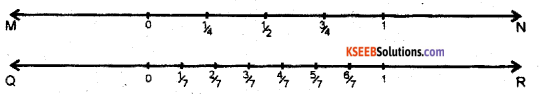$$\frac{2}{7}$$ is closer to $$\frac{1}{4}, \frac{3}{7}$$ is closer to $$\frac{1}{2}$$
$$\frac{5}{7}$$ is closer to $$\frac{3}{4}$$
Estimate the degree of closeness of the fractions $$\frac{2}{9}, \frac{4}{9}, \frac{5}{9}$$ and $$\frac{7}{9}$$ to $$\frac{1}{4}, \frac{1}{2}$$ and $$\frac{3}{4}$$ .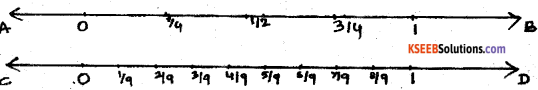$$\frac{2}{9}$$ is closer to $$\frac{1}{4}, \frac{4}{9}$$ is closer to $$\frac{1}{2}$$
$$\frac{5}{9}$$ is closer to $$\frac{1}{2}, \frac{7}{9}$$ is closer to $$\frac{3}{4}$$